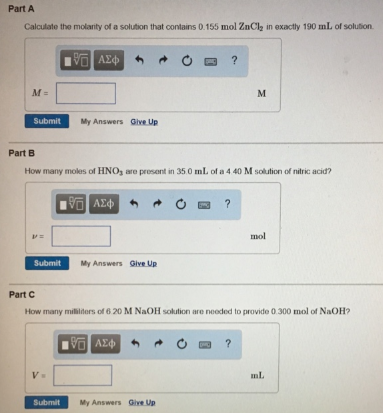# Problem: Part A Calculate the molarity of a solution that contains 0.155 mol ZnCl2 in exactly 190 mL of solution. Part B How many moles of HNO3 are present in 35.0 mL of a 4.40 M solution of nitric acid? Part C How many mililiters of 6.20 M NaOH solution are needed to provide 0.300 mol of NaOH?

###### FREE Expert Solution
79% (50 ratings)###### Problem Details

Part A

Calculate the molarity of a solution that contains 0.155 mol ZnCl2 in exactly 190 mL of solution.

Part B

How many moles of HNO3 are present in 35.0 mL of a 4.40 M solution of nitric acid?

Part C

How many mililiters of 6.20 M NaOH solution are needed to provide 0.300 mol of NaOH?